# Fuzzy topology

lattice-valued topology, point-set lattice-theoretic topology, poslat topology

A branch of mathematics encompassing any sort of topology using lattice-valued subsets. The following description is based on the standardization of this discipline undertaken in [a9], especially [a10], [a11]; much additional information is given in the references below.

Let $X$ be a set and $( L , \leq , \otimes )$ any complete quasi-monoidal lattice (a cqml; i.e., $( L , \leq )$ is a complete lattice with bottom element $\perp$ and top element $\top$, and the tensor product $\otimes : L \times L \rightarrow L$ is isotone in both arguments with $\top \otimes \top = \top$). Examples of complete quasi-monoidal lattices are:

complete lattices with $\otimes = \wedge$ (binary meet);

$L = [ 0,1 ]$ with $\otimes$ any of the $t$-norms $T _ { \operatorname { min } } ( a , b ) = a \wedge b$, $T _ { \text { prod } } ( a , b ) = a \cdot b$, or $T _ { m } ( a , b ) = ( a + b - 1 ) \vee 0$; or

$L = [ 0,1 ] \times [ 0,1 ]$ with $\otimes$ any of $T_{\text{min}} \times T_{\text{prod}}$, $T _ { \operatorname{prod} } \times T _ { m }$, etc.

$L$-subsets of $X$ comprise the $L$-powerset $L ^ { X } = \{ a : X \rightarrow L , a \ \text {a function } \}$, a complete quasi-monoidal lattice via the lifting of the structure of $L$. A subfamily $\tau \subset L ^ { X }$ is an $L$-topology on $X$, and $( X , \tau )$ is an $L$-topological space, if $\tau$ is closed under $\otimes$ and arbitrary $\vee$ and contains the constant mapping $\underline{ \top }$. A function ${\cal T} : L ^ { X } \rightarrow L$ is an $L$-fuzzy topology on $X$, and $( X , \mathcal{T} )$ is an $L$-fuzzy topological space, if $\mathcal{T}$ satisfies (reading $\forall$ as "for each" ):

1) $\forall$ set $J$, $\forall \{ u_j : j \in J \} \subset L ^ { X }$,

\begin{equation*} \bigwedge _ { j \in J } {\cal T} ( u _ { j } ) \leq {\cal T} \left( \underset { j \in J } { \vee } u _ { j } \right). \end{equation*}

2) $\forall$ two-element set $J$, $\forall \{ u_j : j \in J \} \subset L ^ { X }$,

\begin{equation*} \bigotimes _ { j \in J } \mathcal{T} ( u _ { j } ) \leq \mathcal{T} \left( \bigotimes _ { j \in J } u _ { j } \right) . \end{equation*}

3) $\mathcal{T} ( \underline { \top } ) = \top$.

The member $\mathcal{T} ( u )$ of $L$ is interpreted as the "degree of openness" of $u$.

Important examples of $L$-topological and $L$-fuzzy topological spaces can be found in [a5], Chap. 11; [a6], Kubiak's paper; [a8]; [a9], Chaps. 6, 8, 10; [a9], Chap. 7, Sect. 2.15–2.16; [a10], Sect. 7; [a11], Sect. 7.

For a complete quasi-monoidal lattice $L$ and function $f : X \rightarrow Y$, one defines the powerset operators $f _ { L } ^ { \rightarrow } : L ^ { X } \rightarrow L ^ { Y }$ (the image operator) and $f _ { L } ^ { \leftarrow } : L ^ { Y } \rightarrow L ^ { X }$ (the pre-image operator) by

\begin{equation*} f _ { L } ^ { \rightarrow } ( a ) ( y ) = \vee \{ a ( x ) : f ( x ) = y \}, \end{equation*}

\begin{equation*} f _ { L } ^ { \leftarrow } ( b ) = b \circ f. \end{equation*}

It is well-known that $f _ { L } ^ {\rightarrow} \dashv f _ { L } ^ { \leftarrow }$ and that these operators generalize the traditional operators $f^\rightarrow$ and $f ^ { \leftarrow }$. Given $L$-topological spaces $( X , \tau )$ and $( Y , \sigma )$, a mapping $f : X \rightarrow Y$ is $L$-continuous from $( X , \tau )$ to $( Y , \sigma )$ if $f | _ { \sigma } ^ { \leftarrow } : \tau \leftarrow \sigma$; and given $L$-fuzzy topological spaces $( X , \mathcal{T} )$ and $( Y , \mathcal{S} )$, a mapping $f : X \rightarrow Y$ is $L$-fuzzy continuous from $( X , \mathcal{T} )$ to $( Y , \mathcal{S} )$ if ${\cal T} \circ f ^ { \leftarrow } \geq \cal S$ on $L ^ { Y }$. The category $L$-$\mathbf{TOP}$ comprises $L$-topological spaces, $L$-continuous mappings, and the composition and identities from the category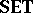(cf. also Sets, category of); and the category $L$-$\textbf{FTOP}$ comprises $L$-fuzzy topological spaces, $L$-fuzzy continuous mappings, and the composition and identities from the category. It is a theorem that for all complete quasi-monoidal lattices $L$, the categories $L$-$\mathbf{TOP}$ and $L$-$\textbf{FTOP}$ are topological categories over, in the sense of [a3] and [a11], Sect. 1, and hence topological constructs.

The above briefly describes "fixed-basis topology" — topology where the complete quasi-monoidal lattice $L$, viewed as the lattice-theoretic base of powersets $L ^ { X }$ and spaces $( X , \tau )$ or $( X , \mathcal{T} )$, is fixed relative to the spaces and mappings of the category $L$-$\mathbf{TOP}$ or $L$-$\textbf{FTOP}$. "Variable-basis topology" permits the base to change within a category, so that each space has its own lattice-theoretic base.

To outline variable-basis topology, note that all complete quasi-monoidal lattices form a category,, in which morphisms are mappings preserving $\otimes$, arbitrary $\vee$, and $\top$; and also note that $\mathbf{FRM}$ and $\textbf{SFRM}$ embed into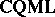. One then considers, with objects the same as those of, now called localic quasi-monoidal lattices, but morphisms reversed from those of; and one notes that $\mathbf{LOC}$ and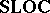embed into.

Now, let. The category $\mathbf{C}$-$\mathbf{TOP}$ for variable-basis topology and the category $\mathbf{C}$-$\textbf{FTOP}$ for variable-basis fuzzy topology are both "concrete" categories over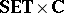as a "ground" or "base" category. For amorphism $( f , \phi ) : ( X , L ) \rightarrow ( Y , M )$, the pre-image operator $( f , \phi ) ^ { \leftarrow } : L ^ { X } \leftarrow M ^ { Y }$ is defined by $( f , \phi ) ^ { \leftarrow } ( b ) = \phi ^ { \text{op} } \circ b \circ f$. An image operator $( f , \phi ) ^ { \rightarrow }$ is also available which, if $\phi ^ { \operatorname{op} }$ preserves arbitrary $\wedge$, satisfies $( f , \phi )^{ \rightarrow} \dashv ( f , \phi )^{ \leftarrow}$; and if $L = M , \phi ^ { \operatorname{op} } = \operatorname{id} _ { L }$, these operators reduce to their fixed-basis counterparts.

Data for the category $\mathbf{C}$-$\mathbf{TOP}$ include:

objects are topological spaces $( X , L , \tau )$ (cf. also Topological space), where $( X , L ) \in | \mathbf{SET} \times \mathbf{C}|$ and $( X , \tau ) \in | L \square \mathbf{TOP} |$;

morphisms are continuous mappings

\begin{equation*} ( f , \phi ) : ( X , L , \tau ) \rightarrow ( Y , M , \sigma ) \end{equation*}

(cf. also Continuous function), where $( f , \phi ) : ( X , L ) \rightarrow ( Y , M )$ is inand $( f , \phi ) ^ { \leftarrow } | _ { \sigma } : \tau \leftarrow \sigma$.

Data for the category $\mathbf{C}$-$\textbf{FTOP}$ include:

objects are fuzzy topological spaces $( X , L , \mathcal{T} )$, where $( X , L ) \in | \mathbf{SET} \times \mathbf{C}|$ and $( X , \mathcal{T} ) \in | L \square \mathbf{FTOP}|$;

morphisms are fuzzy continuous mappings $( f , \phi ) : ( X , L , \mathcal{T} ) \rightarrow ( Y , M , \mathcal{S} )$, where $( f , \phi ) : ( X , L ) \rightarrow ( Y , M )$ is inand $\mathcal{T} \circ ( f , \phi ) ^ { \leftarrow } \geq \phi ^ { \operatorname{op} } \circ \mathcal{S}$ on $M ^ { Y }$.

In both categories, compositions and identities are those of.

It is a theorem that for all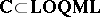, $\mathbf{C}$-$\mathbf{TOP}$ and $\mathbf{C}$-$\textbf{FTOP}$ are topological over the groundin the sense of [a3] and [a11], Sect. 1. Further, these frameworks unify all the fixed-basis categories for topology given above and hence unify all important examples (referenced above) over different lattice-theoretic bases (e.g. two fuzzy real lines $\mathbf{R} ( L )$ and $\mathbf{R} ( M )$). Moreover, all purely lattice-theoretic or point-free approaches to topology — locales, topological molecular lattices, uniform lattices, etc. (see [a1], [a2], [a8]) — categorically embed into $\mathbf{C}$-$\mathbf{TOP}$ or $\mathbf{C}$-$\textbf{FTOP}$ (for appropriate $\mathbf{C}$) as subcategories of singleton spaces; e.g. $\mathbf{LOC}$ embeds as singleton spaces into $\mathbf{LOC}$-$\mathbf{TOP}$. Thus, the variable-basis approach categorically unifies topology and fuzzy topology as a discipline.

How to Cite This Entry:
Fuzzy topology. Encyclopedia of Mathematics. URL: http://encyclopediaofmath.org/index.php?title=Fuzzy_topology&oldid=50746
This article was adapted from an original article by Stephen E. Rodabaugh (originator), which appeared in Encyclopedia of Mathematics - ISBN 1402006098. See original article